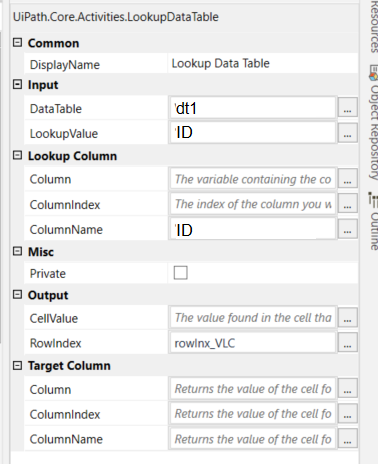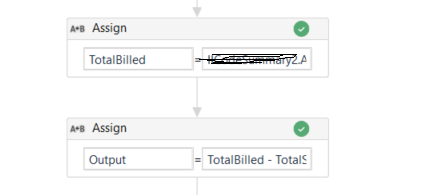# Can't figure out Lookup Datatable and some functions :(

Hi everyone, I am trying to do billing reconciliation. Here is what I have and what I am trying to achieve. Do note that my fields that contains dollar amounts are strings and I also face alot of difficult with the error: Implicit conversion disallowed from string to double, etc.

dt1
ID, AmtIssued
ABC1234, \$500
DEF5678, \$800

dt2
ID, AmtBilled, Outstanding
ABC1234, \$100
ABC1234, \$200
DEF5678, \$600

Outcome: The column Outstanding = AmtBilled - AmtIssued

So the outcome I should have is:
ID, AmtBilled, Outstanding
ABC1234, \$100, -\$300
ABC1234, \$200, -\$300
DEF5678, \$600, -\$200

Where the \$300 is derived by taking (\$100+\$200) - \$500

What I have done is

Assigned TotalAmt = dt2.AsEnumerable.Select(Function (x) x(“ID”).toString).Distinct().toArray

then I created a For Each loop:

My Lookup DataTable input:Moving on,

Where TotalSum = convert.ToDouble(dt1.Rows(rowInx_VLC).Item(“AmtIssued”).ToString)

ThenWhere TotalBilled = d2.AsEnumerable.Sum(Function(a)Convert.ToDouble(a(“AmtBilled”).ToString))

Output = TotalBilled - TotalSum

Errors that I’m facing:

• Output is being calculated incorrectly
*After I get Output, I’m not sure how to write it into the Outstanding column in dt2

I hope the above is clear. Please let me know if you require any clarification. I would really appreciate the help!
Also, due to the permissions settings, I’m not able to download any xaml files into my laptop so I hope that any explanation can be done in text or via screenshots. Thank you!

Hi,

If I understood your requirement right, the above should be \$-200, right ?

``````dictAmtIssued = dt1.AsEnumerable.ToDictionary(Function(r) r("ID").ToString,Function(r) Double.Parse(System.Text.RegularExpressions.Regex.Match(r("AmtIssued").ToString,"[-\d.,]+").Value))

dictAmtBilledSum = dt2.AsEnumerable.GroupBy(Function(r) r("ID").ToString).ToDictionary(Function(g) g.Key,Function(g) g.Sum(Function(r) Double.Parse(System.Text.RegularExpressions.Regex.Match(r("AmtBilled").ToString,"[-\d.,]+").Value) ))
``````

Then

``````dt2 = dt2.AsEnumerable.Select(Function(r) dt2.Clone.LoadDataRow({r("ID"),r("AmtBilled"),"\$"+(dictAmtBilledSum(r("ID").ToString)-dictAmtIssued(r("ID").ToString)).ToString},False)).CopyToDataTable()
``````

Note : dictAmtIssued and dictAmtBilledsum are `Dictionary<string,double>` type

Regards,